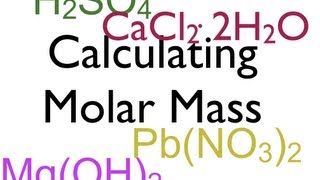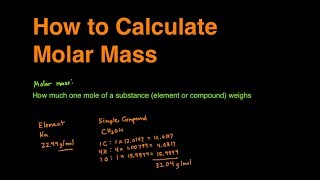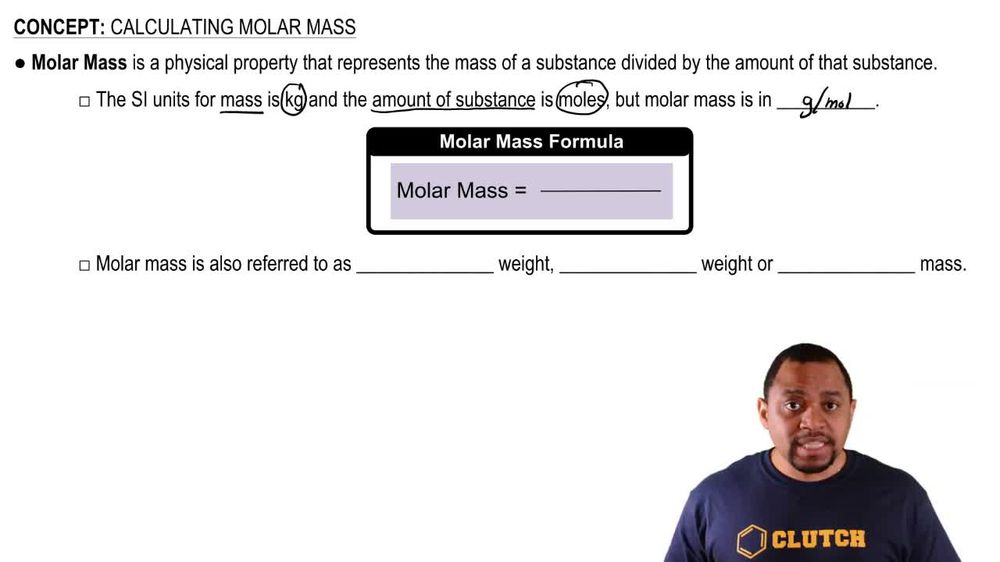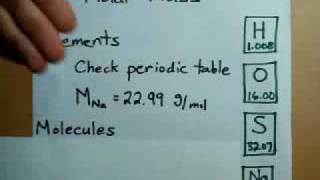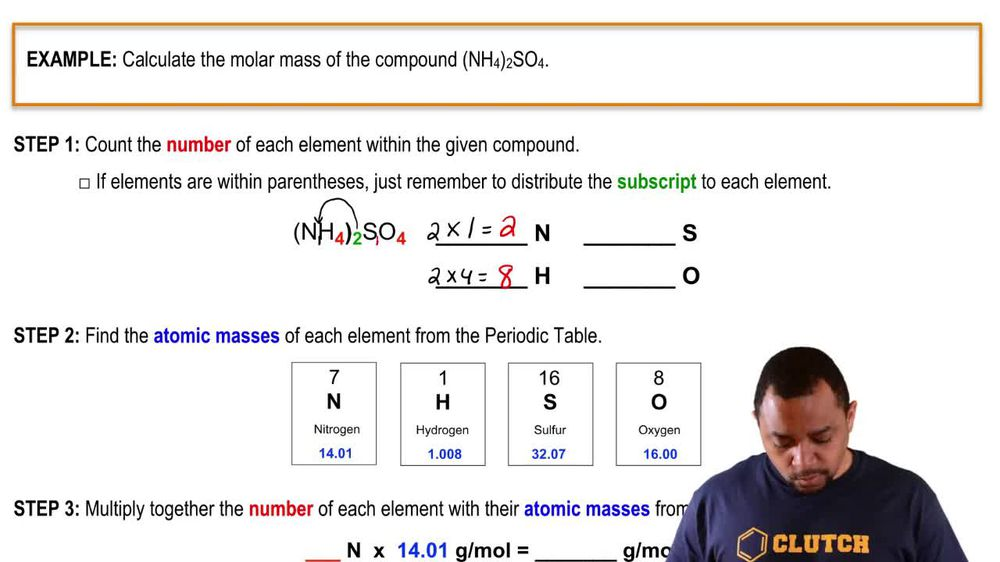Start typing, then use the up and down arrows to select an option from the list.
1. 2. Atoms & Elements2. Calculating Molar Mass
Problem

# Cytochrome c is an iron-containing enzyme found in the cells of all aerobic organisms. If cytochrome c is 0.43% Fe by mass, what is its minimum molecular weight?

Relevant Solution1m
Play a video:
Hey everyone, we're told that heem is an iron containing complex that binds oxygen to blood. Analysis of him shows that it is 9.06% iron by mass, calculate the molar mass of him. To answer this question, we need to know the formula mass percent is equal to the mass of our element divided by the mass of our compound. Now, if we plug in our values, we have our mass percent, which is 9.06%. When we convert this into decimal form, it will be 0.0906. And this is going to be equal to the mass of our element, which is 55.85 g per mole, which can be found in our periodic table. And this is the molar mass of iron. And we're going to divide this by the mass of our compound which we can label as X. So solving for X, we're going to multiply X onto both sides And we get x times 0.0906 equals 55. g per mole. Now when we divide 0.0906 onto both sides, We end up with an x. 616.4 g per mole. And this is going to be our final answer and our molar mass of him. Now I hope that made sense. And let us know if you have any questions